# NCERT Solutions for Class 7 Maths Chapter 14 Symmetry

NCERT Solutions for Class 7 Maths Chapter 14 Symmetry

### NCERT Solutions for Class 7 Maths Chapter 14 Symmetry Ex 14.1

NCERT Solutions for Class 7 Maths Chapter 14 Symmetry Exercise 14.1
Ex 14.1 Class 7 Maths Question 1.
Copy the figures with punched holes and find the axis of symmetry for the following:Solution:
The axis of symmetry is shown by following line.Ex 14.1 Class 7 Maths Question 2.
Give the line(s) of symmetry, find the other hole(s):Solution:Ex 14.1 Class 7 Maths Question 3.
In the following figures, the mirror line (i.e., the line of symmetry) is given as dotted line. Complete each figure performing reflection in the dotted (mirror) line. (You might perhaps place a mirror along the dotted line and look into the mirror for the image). Are you able to recall the name of the figure you complete?Solution:
Following are the complete figures.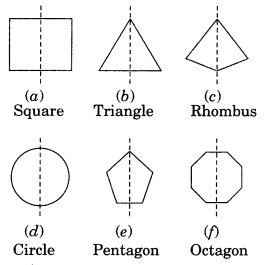Ex 14.1 Class 7 Maths Question 4.
The following figures have more than one line of symmetry. Such figures are said to have multiple lines of symmetry.Identify multiple lines of symmetry, if any, in each of the following figures:Solution:
Here, figure (6), (d), (e), (g) and (h) are the multiple lines of symmetry.

Ex 14.1 Class 7 Maths Question 5.
Copy the figure given here.
Take any one diagonal as a line of symmetry and shade a few more squares to make the figure symmetric about a diagonal. Is there more than one way to do that? Will the figure be symmetric about both the diagonals?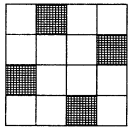Solution:
(i) Let us take a diagonal as the axis of symmetry and shade the square as shown in the figure.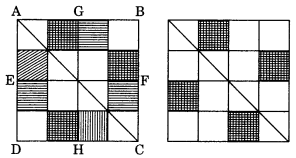(ii) Yes, there are more than the line of symmetry i.e. BD, EF and GH.
(iii) Yes, the figure is symmetric about both the diagonals.

Ex 14.1 Class 7 Maths Question 6.
Copy the diagram and complete each shape to be symmetric about the mirror line(s).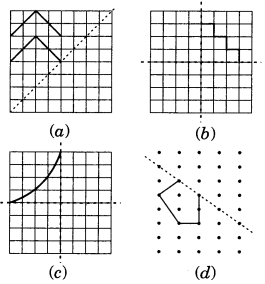Solution:Ex 14.1 Class 7 Maths Question 7.
State the number of lines of symmetry for the following figures:
(а) An equilateral triangle
(b) An isosceles triangle
(c) A scalene triangle
(d) A square
(e) A rectangle
(f) A rhombus
(g) A parallelogram
(i) A regular hexagon
(j) A circle
Solution:

 Figure Number of lines of symmetry (a) An equilateral triangle 3 (6) An isosceles triangle 1 (c) A scalene triangle 0 (d) A square 4 (e) A rectangle 2 (f) A rhombus 2 (g) A parallelogram 0 (h) A quadrilateral 0 (i) A regular hexagon 6 (j) A circle Infinite

Ex 14.1 Class 7 Maths Question 8.
What letters of the English alphabet have reflectional symmetry (i.e. symmetry related to mirror reflection) about
(а) a vertical mirror
(b) a horizontal mirror
(c) both horizontal and vertical mirrors.
Solution:
(a) Alphabet of vertical mirror reflection symmetry are
A, H, I, M, O, T, U, V, W, X, Y
(b) Alphabet of horizontal mirror reflection symmetry are:
B, C, D, E, H, I, K, O, X
(c) Alphabet of both horizontal and vertical mirror reflection symmetry are:
H, I, O, X.

Ex 14.1 Class 7 Maths Question 9.
Give three examples of shapes with no line of symmetry.
Solution:
Example 1: Scalene triangle has no line of symmetry.
Example 2: Quadrilateral has no line of symmetry.
Example 3: Alphabet R has no line of symmetry.

Ex 14.1 Class 7 Maths Question 10.
What other name can you give of the line of symmetry of
(a) an isosceles triangle?
(b) a circle?
Solution:
(a) Median of an isosceles triangle is its line of symmetry.
(b) Diameter of a circle is its line of symmetry.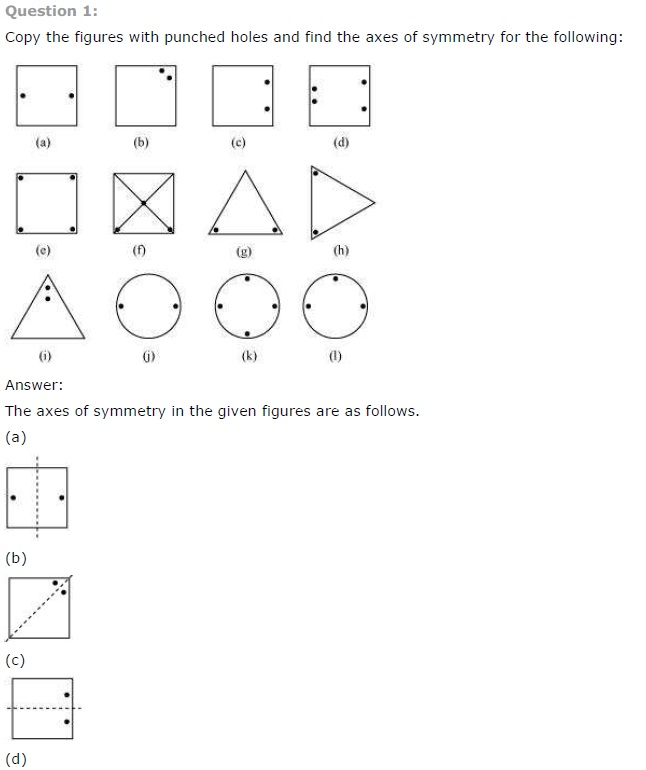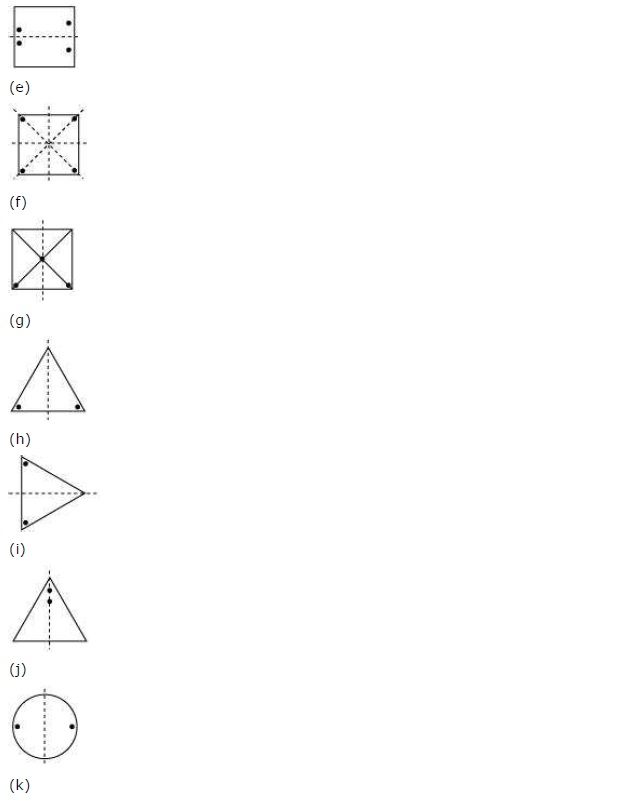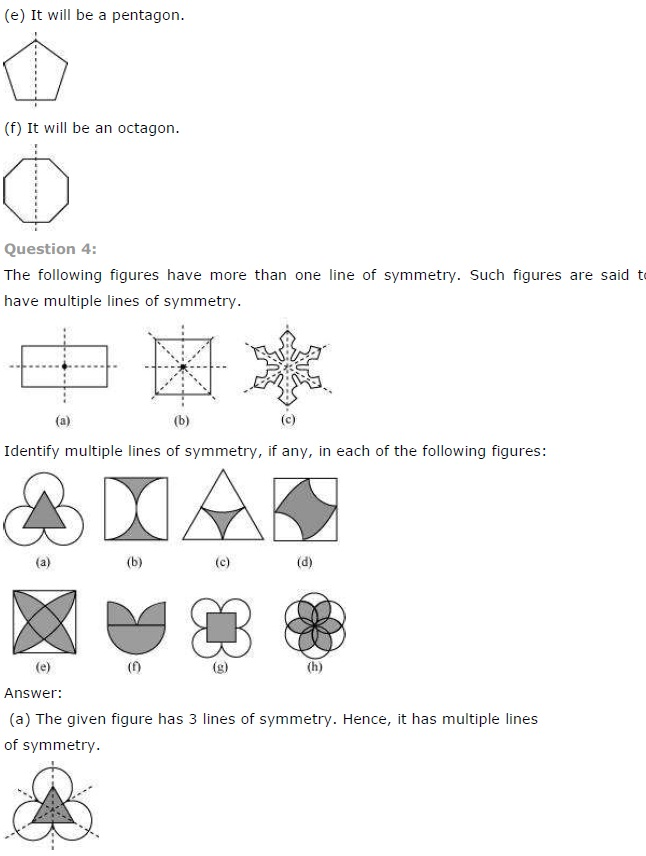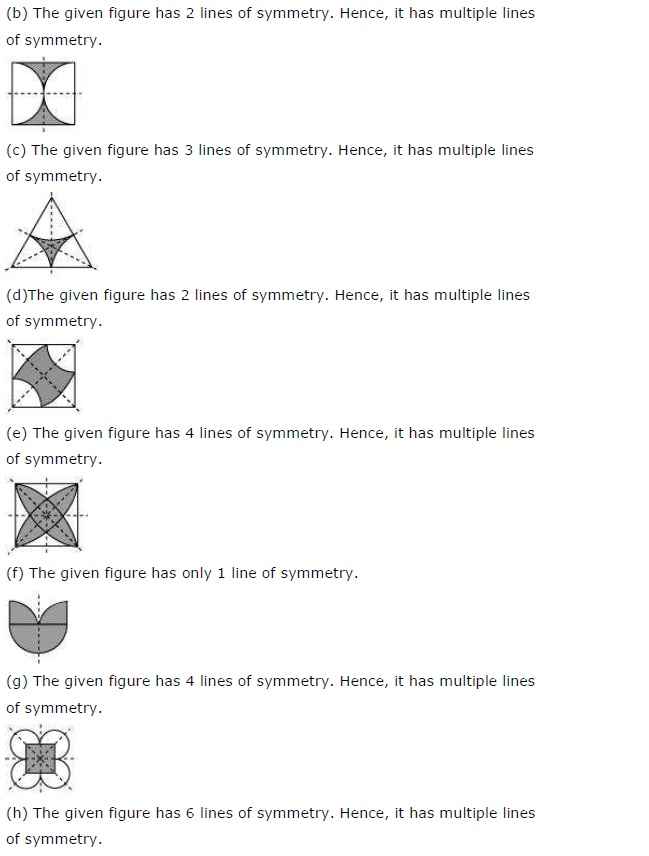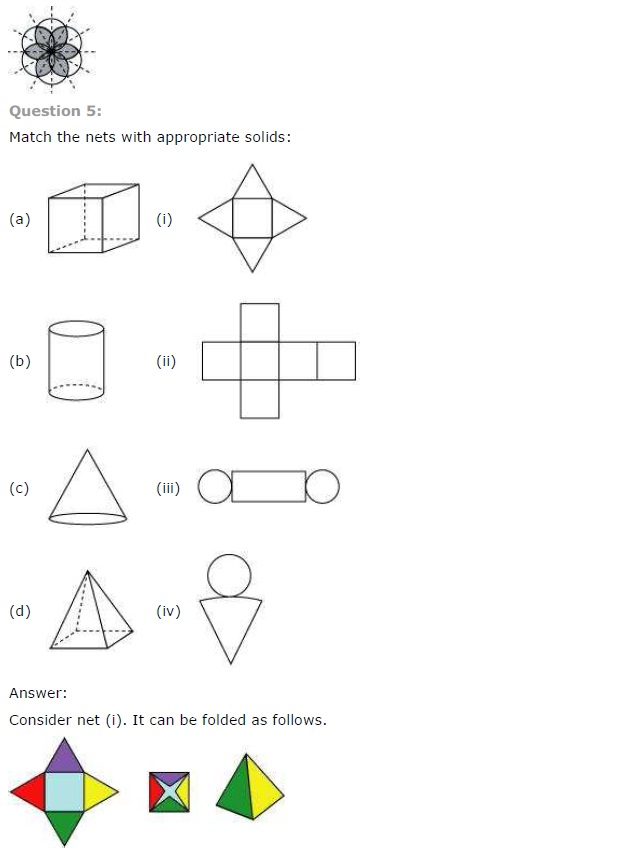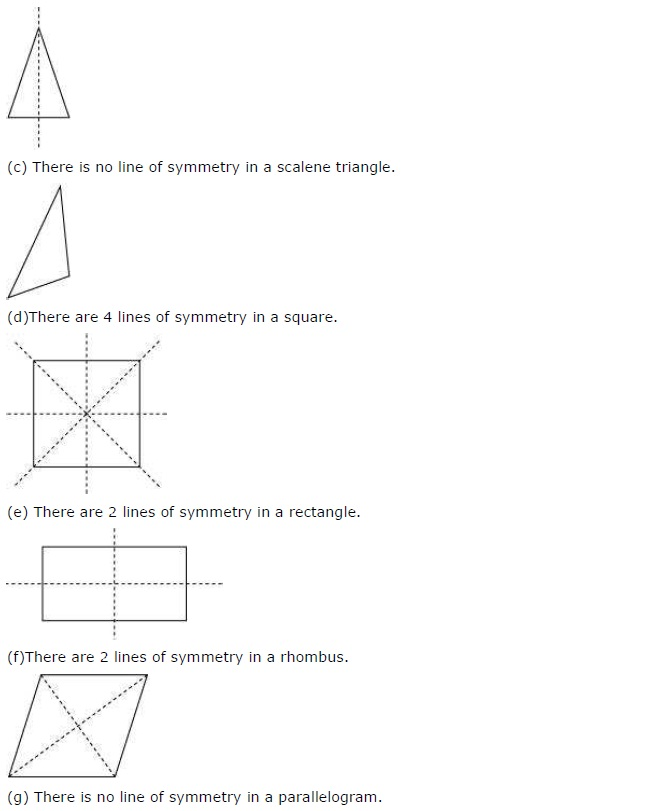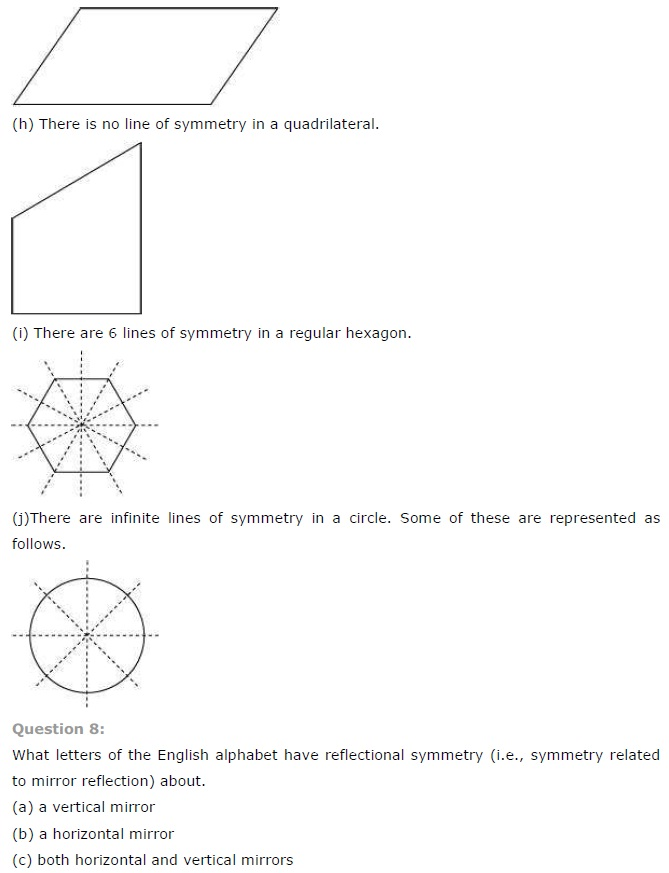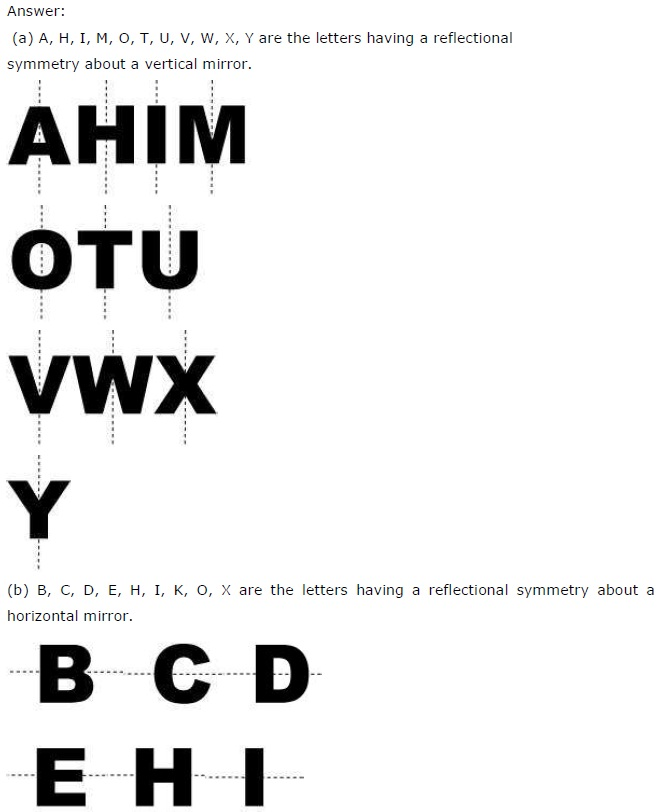## SabDekho

The Complete Educational Website